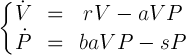# Predators and Prey: the Lotka–Volterra model

The first model describing the interaction between two populations in the ecosystem is the Lotka–Volterra model. It applies to a predator-prey system (population of predators feeds on the individuals of the prey species). The model was proposed in 1920s in parallel by Vito Volterra as a model of a population, and by Alfred Lotka as a model of a chain of chemical reactions. Volterra proposed this model to explain the phenomenon that was observed after the First World War. It was noted that the population of predatory fish in the Adriatic Sea had increased. This was considered a paradox, because it would seem that all species should be negatively affected by the war. Volterra based on his model showed that the increase in the number of predators was natural because fishing was stopped during the war, and thus the population of predators could return to its natural state.

The model takes the following assumptions:

• In the environment there are only two species: the prey and the predators.
• If there are no predators in the environment, prey have favorable conditions (i.e., the are no additional limitations in the environment) and their population increases exponentially (according to the Malthus model).
• If there are no prey in the environment, the predators do not have food, which means lack of energy necessary for survival and reproduction, resulting in extinction of the species (population size decreases exponentially).
• Assuming that both species exist in the environment, some part of prey are killed by predators. Food provides the energy necessary for predators for reproduction, and thus the size of their population grows.
• Hunting is only possible in case of direct contact. Individuals move randomly, so the number of contacts is proportional to the number of individuals of both species.
• A specific spatial distribution of individuals in the environment is not taken into account. We consider only the average density of individuals.

## Lotka–Volterra simulation

Select language of the program:

#### Program description

The above multi-agent simulation is inspired by the Lotka–Volterra model. The simulation is not a perfect equivalent of the model: the model has a continuous nature, and besides, the simulation takes into account the position of specimen in the environment. In the considered model, two species interact with each other: prey (rabbits) and predators (foxes). Individuals move in a random way and are visualized by a green and a red color.

Default parameters allow to observe oscillation of population cardinality for rabbits and foxes. This can be explained in simple terms: increasing the number of rabbits results in the short time increase in the number of foxes. The population size grows due to the availability of food. But after some time, there is not enough food for the entire population of foxes and they start dying. In turn, this will affect the regeneration of rabbits and the cycle starts over.

The size and variability over time (i.e., population dynamics) can be seen on the charts. The first one shows the so-called phase portrait of the system of equations. The axes of the coordinate system correspond to the cardinality of the population of both species and points illustrate inter-related population sizes for several recent steps of the simulation. The second chart shows the dependence of the number of individuals of each species, depending on time (which is associated with the solution of equations system of the L-V model – see below). Axes describing sizes of populations have relative units, e.g. 50% means that a half of the environment is occupied by a given population.

Tips for using the program:

#### Simulation parameters

• World size determines the size of the simulation environment.
• Initial % Rabbits i Initial % Foxes refer to initial percentage of population occupancy in the environment.
• P(R Breed) corresponds to the parameter r from L-V system, which is described below.
• P(F Breed) corresponds to the parameter b from L-V system.
• P(F Die) corresponds to the parameter s from L-V system.
• P(F Effectiveness) corresponds to the parameter a from L-V system.

The parameters that are equivalent to the L-V system of equations are interpreted as the probability of occurrence of relevant events in subsequent iterations of the simulation. You can experiment with the parameters during the simulation to observe how they influence the size of populations of rabbits and foxes. Try to set parameters that lead to the extinction of one of the populations.

#### Running the simulation

The Start button starts the simulation. Simulation speed can be controlled by the appropriate slider. During simulation, it is possible to change some parameters and their impact on the ecosystem can be observed live in the environment and the charts. The Reset button causes random initialization of the environment, according to the World size, Initial % Rabbits and Initial % Foxes.

### Theory

Let us denote by

V(t) – number (density) of prey at the moment t;
P(t) – number (density) of predators at the moment t.

The system of equations together with the assumptions given above is called the Lotka–Volterra model (L-V) or the predator–prey model. For simplicity, the independent variable t is omitted. The equations arewhere

• r is the coefficient of reproduction of the prey species;
• aVP means biomass of hunted preys, where a is the hunting efficiency coefficient, which determines what part of meetings between preys and predators ends up with hunting down of prey;
• b is a part of the energy obtained by predators from hunting and used for reproduction (the conversion of hunted prey biomass);
• s is the mortality rate of the predator species.

It turns out that for non-negative initial data, there exist solutions that are unambiguous and non-negative – therefore they have a biological meaning. The solution of this system of equations consists of two periodic functions shifted in phase.

 Program and text: Łukasz Idkowiak porting to javascript: Magdalena Waligórska Mentor: Maciej Komosinski